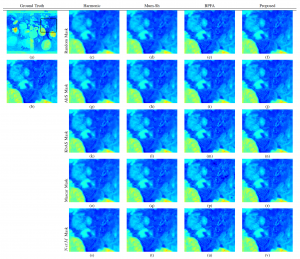Pipeline for adaptive sampling mask generation utilizing CNNs. Given an RGB input image z, the binary sampling mask m will be generated by NetM. The corrupted image z’ is obtained by applying the binary sampling mask m on the RGB image z. Finally, the restorted RGB image is estimated by NetE.

### Project Description

This paper presents an adaptive image sampling algorithm based on Deep Learning (DL). It consists of an adaptive sampling mask generation network which is jointly trained with an image inpainting network. The sampling rate is controlled by the mask generation network, and a binarization strategy is investigated to make the sampling mask binary. In addition to the image sampling and reconstruction process, we show how it can be extended and used to speed up raster scanning such as the X-Ray fluorescence (XRF) image scanning process. The experiments show that the proposed adaptive sampling algorithm is able to effectively sample the image and achieve a better reconstruction accuracy than that of existing methods.

Preprint

### Codes

Github Repository

### ImagesNetwork architecture for the mask generation network (NetM). Residual blocks with skip connections are used in the binary sampling mask network.One application of the proposed binary sampling algorithm is to speed up the raster sampling process of some imaging application, such as XRF imaging. The proposed pipeline for the XRF image inpainting utilizing an adaptive sampling mask is shown in this figure. The binary adaptive sampling mask is generated based on the RGB image of the scan target. Then, the XRF scanner sampled the target object based on the binary sampling mask. Finally, the subsampled XRF image and the RGB image are fused to reconstruct the fully sampled XRF image.Visual Comparison of the reconstructed images using random, AIrS, KbAS, and NetM sampling masks at sampling rate c = 0.2. The first column is the input test image and the second column is the sampling mask, either random, AIrS, KbAS, or NetM, the third column is the sampled image obtained by the sampling mask, and the rest of the columns are the reconstruction results of NetE Inpainting, Harmonic Inpainting, Mumford-Shah Inpainting, and BPFA inpainting respectively.Visualization of sampling and inpainting result of the “Bloemen en insecten” painting. (a) original RGB image with red bounding box. (b) region inside the bounding box of (a) for visualization purposes. (c), (g), (k), (o) and (s) random, AIrS, KbAS, Mascar and NetM sampling masks respectively. (d), (h), (l), (p) and (t) reconstruction results of each sampling mask using Harmonic algorithms. (e), (i), (m), (q) and (u) reconstruction results of each sampling mask using Mumford-Shah algorithm. (f), (j), (n), (r) and (v) reconstruction results of each sampling mask using BPFA algorithm. Computation time of each sampling mask and PSNR of the entirety of each reconstructed images are also shown.Visualization of inpainting results on the “Bloemen en insecten” data when c = 0.2. Channel #16 related to the Pb L XRF emission line is selected. (a) ground truth XRF image with black bounding box. (b) region inside the bounding box of (a), shown for visual comparison purposes. The sampling masks for random, AIrS, KbAS, Mascar and NetM are the same as the sampling masks in Figures 9 (c), (g), (k), (o) and (s) respectively. (c)-(v) reconstruction results of different inpainting algorithms for different sampled XRF image within the same region of (a) as (b).